# Insertion in AVL Trees

## Introduction

A tree is a hierarchical data structure where data is stored in nodes interconnected via some edges. A General Tree can have any number of nodes. A Binary Tree is a type of tree where each node has at max two child nodes. A Binary Search Tree or BST is a binary tree where the left child is less than the right child. If the difference between the height of the left subtree and the right subtree of a BST is not more than one then, that tree is called Balanced BST. An AVL tree is a binary search tree that is height-balanced for each node. The following Venn diagram is a visual aid for better understanding.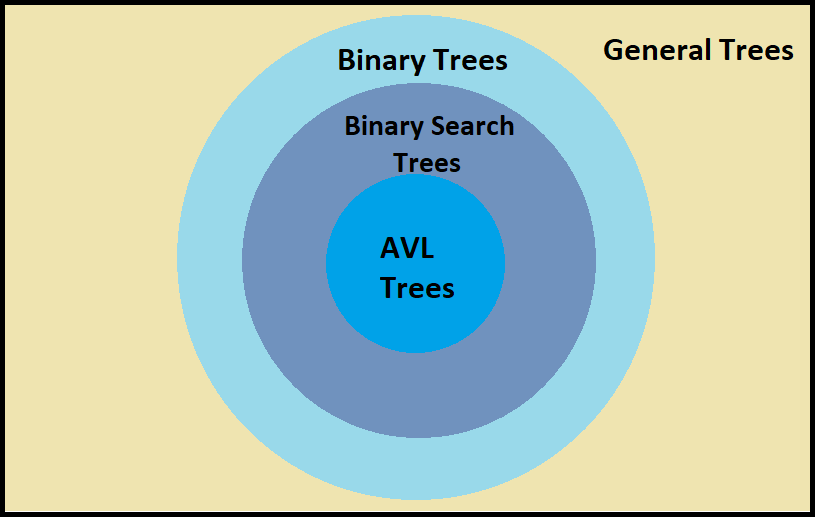Learning everything about AVL trees at once is a tedious task. So to ease the pace of learning the whole blog is divided into 3 different blog series. The content of the three blogs are:

1. Introduction to AVL Trees
2. Insertion in AVL Trees
3. Deletion in AVL Trees

## AVL Trees

AVL trees are self-balancing BST where the difference between the height of the left and right subtrees is either -1,0 or 1.

AVL trees are binary search trees in which:

1. The height of each sub-trees differs by at most one.
2. Every sub-tree is an AVL tree.

For insertion in AVL trees, we first place a node into the appropriate place in the binary search tree. After performing insertion, we calculate the Balance Factor (BF)  for every node and check whether it is equal to -1,0,1 or not. If it is not equal, we perform “rotations” on the tree to balance it.

To calculate the Balance Factor (BF) of a node, we simply find the difference between the heights of the left subtree and right subtree, and the difference must be -1,0,1. Thus,

Balance Factor (BF) = (height of left subtree - height of right subtree) ∈ {-1,0,1}.

At any point, only three nodes can be taken for any kind of rotation. Keeping this in mind, the four types of rotations are as follows:

## Left-Left (LL) rotation

Consider the input sequence {30,20,10}. If we insert these values in our BST, then the BST formed will look  like fig: 1(a)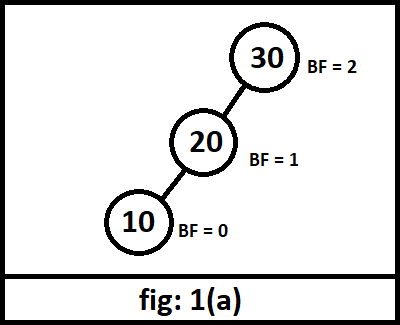It is clear from the illustration that the tree becomes LL-imbalanced as it BF = 2 at the root node, which is not acceptable. To make it balanced, we will perform LL rotation. After completing LL rotation, our tree will look like fig: 1(b).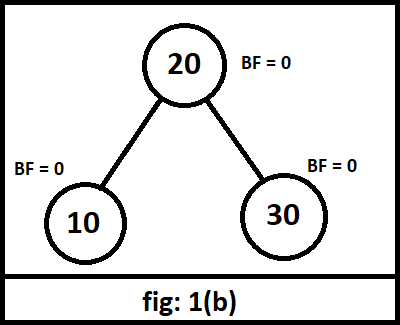## Left-Right (LR) rotation

Consider the input sequence {30,10,20}. If we insert these values in our BST, we will get the tree as shown in fig: 2(a).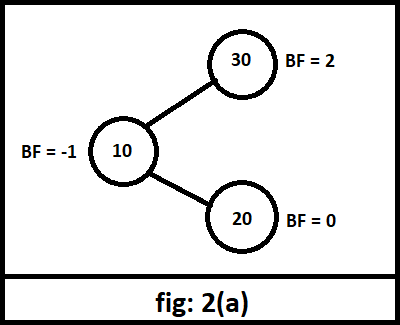Again the tree formed is LR-imbalanced, and we need to balance it by performing LR rotation. We will first rotate about 10, shown in fig: 2(b), and then about 30, shown in fig: 2(c). The point to be noted is that this is a two-step rotation, not a double rotation.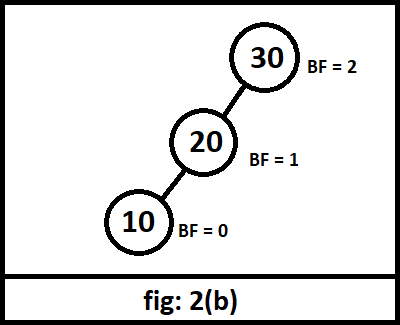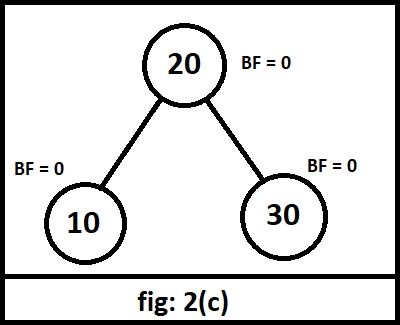## Right-Right (RR) rotation

Consider the input sequence {10,20,30}. If we insert these values into our BST, then we will get the illustration fig: 3(a).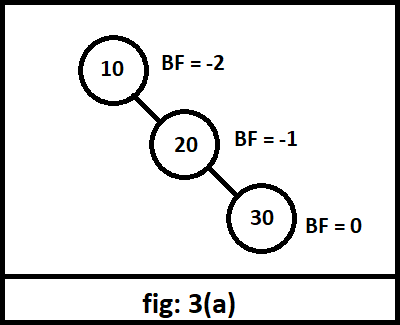The BST obtained has a balance factor of -2 at the root and all the elements on the right subtree. This condition is called RR-imbalance and can be removed by RR rotation, as shown in fig: 3(b).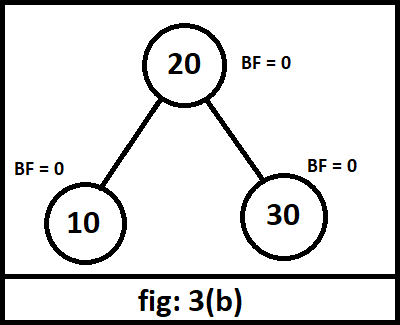## Right-Left (RL) rotation

Consider the input sequence {10,30,20}. The BST formed after inserting these values is shown in fig: 4(a).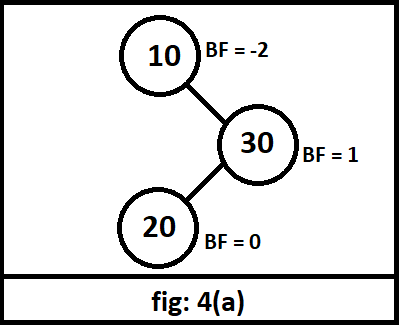The tree so formed is RL-imbalanced because it has a balance factor of -2 at the root node. So, to remove it, we will perform a two-step rotation called RL rotation, as shown in fig: 4(b) and fig: 4(c).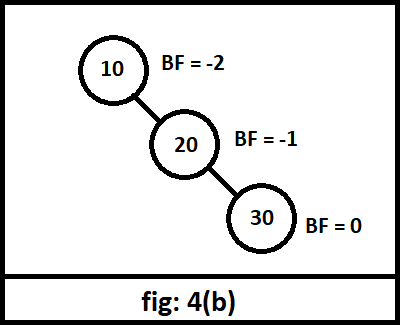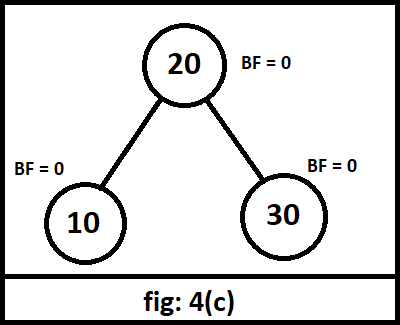## Implementation

In this article, the insertion in the AVL tree is done in C++. Some of the essential functions have been explained below.

## constructor: class AVLtree

Constructor to make our AVL tree with four member variables which are - key (int type) to store the incoming value that has to be added to our tree; *left and *right (AVLtree type) to store the left and right nodes; height (int type) to store the height of out AVL tree.

## function: AVLtree *leftRotate(AVLtree *t1)

This function performs the left rotation operation by taking an argument t1 (AVLtree type) and returns the tree after performing the left rotation. Here again, we take two pointers of type AVLtree - curr (to store the right child of the incoming tree) and t2 (to store the left child of curr node). We change the left child of curr to t1 and the right child of t1 to t2. This rearranges the tree by pulling the nodes towards the left, making the middle node root node and the remaining two nodes the left and right child, respectively. In the end, we change the heights of the subtrees and return the new re-arranged AVL tree.

## function: AVLtree *rightRotate(AVLtree *t1)

This function performs the right rotation operation by taking an argument t1 (AVLtree type) and returns the tree after performing the right rotation. We take two pointer variables of type AVLtree - curr (to store the left child of the incoming tree) and t2 (to store the right child of the curr node). We change the right child of curr to t1 and the left child of t1 to t2. This rearranges the nodes so that in the case of an imbalance, the middle node is made the root node, and the remaining two nodes become the left and the right child, respectively. In the end, we change the heights of both the subtrees and return the new re-arranged AVL tree.

## function: AVLtree *insertNode(AVLtree *tree, int key)

This function performs the insertion in AVL trees by taking two arguments *tree (AVLtree type pointer variable) and key (int type). For performing the insertion in AVL tree we either make a new tree (if there is no tree present) else compare the key value and accordingly place it in our tree. After performing the insertion in AVL tree we calculate the balance factor and perform rotations in case the tree is imbalanced.

## Complete C++ implementation for insertion in AVL Trees

``````#include<iostream>
using namespace std;

class AVLtree
{
public:
int key;
AVLtree *left;
AVLtree *right;
int height;
};

int max(int a, int b)
{
return (a > b)? a : b;
}

int getHeight(AVLtree *tree)
{
if (tree == NULL)
return 0;
return tree->height;
}

AVLtree* newNode(int key)
{
AVLtree* tree = new AVLtree();
tree->key = key;
tree->left = NULL;
tree->right = NULL;
tree->height = 1;
return(tree);
}

AVLtree *leftRotate(AVLtree *t1)
{
AVLtree *curr = t1->right;
AVLtree *t2 = curr->left;

curr->left = t1;
t1->right = t2;

t1->height = max(getHeight(t1->left),
getHeight(t1->right)) + 1;
curr->height = max(getHeight(curr->left),
getHeight(curr->right)) + 1;
return curr;
}

AVLtree *rightRotate(AVLtree *t1)
{
AVLtree *curr = t1->left;
AVLtree *t2 = curr->right;

curr->right = t1;
t1->left = t2;

t1->height = max(getHeight(t1->left),
getHeight(t1->right)) + 1;
curr->height = max(getHeight(curr->left),
getHeight(curr->right)) + 1;

return curr;
}

int getBalance(AVLtree *tree)
{
if (tree == NULL)
return 0;
return getHeight(tree->left) - getHeight(tree->right);
}

AVLtree* insertNode(AVLtree* tree, int key)
{
if (tree == NULL)
return(newNode(key));

if (key < tree->key)
tree->left = insertNode(tree->left, key);
else if (key > tree->key)
tree->right = insertNode(tree->right, key);
else
return tree;

tree->height = 1 + max(getHeight(tree->left),
getHeight(tree->right));

int balance = getBalance(tree);

if (balance > 1 && key < tree->left->key)
return rightRotate(tree);

if (balance < -1 && key > tree->right->key)
return leftRotate(tree);

if (balance > 1 && key > tree->left->key)
{
tree->left = leftRotate(tree->left);
return rightRotate(tree);
}

if (balance < -1 && key < tree->right->key)
{
tree->right = rightRotate(tree->right);
return leftRotate(tree);
}
return tree;
}

void preOrder(AVLtree *root)
{
if(root != NULL)
{
cout << root->key << " ";
preOrder(root->left);
preOrder(root->right);
}
}

// Driver Code
int main()
{
AVLtree *tree = NULL;

tree = insertNode(tree, 10);
tree = insertNode(tree, 20);
tree = insertNode(tree, 30);
tree = insertNode(tree, 40);
tree = insertNode(tree, 50);
tree = insertNode(tree, 25);

cout << "Preorder traversal of the AVL tree is: \t";
preOrder(tree);

return 0;
}
``````

Output

On running the above code with the input sequences {10,20,30,40,50,60}, {60,50,40,30,20,10}, {10,60,30,50,20,40} we get the following output.

The outputs are in preorder traversal (Root, Left child, Right Child).

``````Values added to the AVL tree: 10 20 30 40 50 60
Preorder traversal of the AVL tree is:  40 20 10 30 50 60

*******************************************

Values added to the AVL tree: 60 50 40 30 20 10
Preorder traversal of the AVL tree is:  30 20 10 50 40 60

*******************************************

Values added to the AVL tree: 10 60 30 50 20 40
Preorder traversal of the AVL tree is:  30 10 20 50 40 60``````

The three AVL trees obtained from this execution are shown below: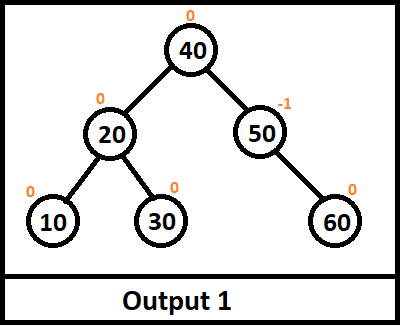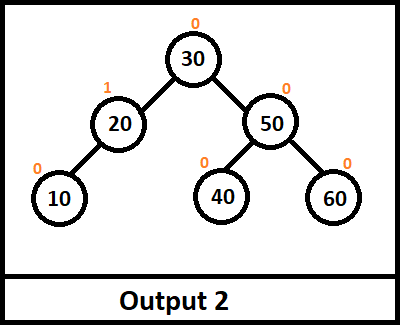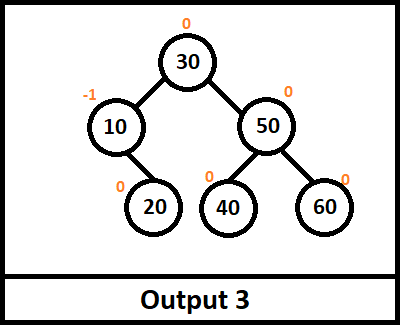## Complexities

### Time complexity

In the above implementation, both the rotations (left and right) take constant time, O(1), as we only change a few pointer values.

While performing insertion in AVL trees, the time taken is the same as a BST which is O(h), where h is the height of the tree. But since our tree is also height balanced, the height is always O(log n). Keeping all these factors in mind, the time complexity of the insert function comes out to be O(log n).

Thus, the overall time complexity (average and worst) of insertion function in an AVL tree is:

T(n) = O(log n)

### Space complexity

The space complexity of an AVL tree is equivalent to the number of nodes present in the tree, which is n. Thus the space complexity comes out to be,

Space complexity = O(n).

Since AVL trees are self-balancing BST where the difference between the height of the left and right subtrees is either -1,0 or 1. Therefore to know how to do insertion operations in an AVL tree you should watch this video tutorial to understand it better.

## FAQs

1. What are AVL trees?
Adelson-Velskii and Landis trees or AVL trees are special binary search trees that are height-balanced for each node. We can perform insertion and deletion operations at any node provided we rearrange the tree if the insertion or deletion causes any imbalance.

2. Why do we perform rotations in an AVL tree?
One of the unique features of AVL trees is that they are self-balancing BST. To make sure that every node is height-balanced, we calculate the balancing factor (BF) and accordingly perform rotations so that the bf value is {-1,0,1} only.

## Key Takeaways

To summarize, this article elaborated on the topic - insertion in AVL trees. It also covered the major four types of rotations that are required for the same.

The blog about AVL Trees is a three-part blog series. This article is the second blog that is concerned with insertion in AVL trees. It is highly recommended to check the Introduction to AVL Trees and Deletion in AVL Trees too to obtain complete knowledge about AVL Trees.

Are you confident enough to perform insertion in AVL trees yourself? Try it out on our CodeStudio Insertion in AVL Tree

Want to know more about Tree data structure? Here’s a great article to help you: The Ultimate Guide To Master Tree Data Structures

Want to crack the interviews to major IT companies? Start your preparation by listening to some of the experiences at Interview Experiences of top tech companies for practice.